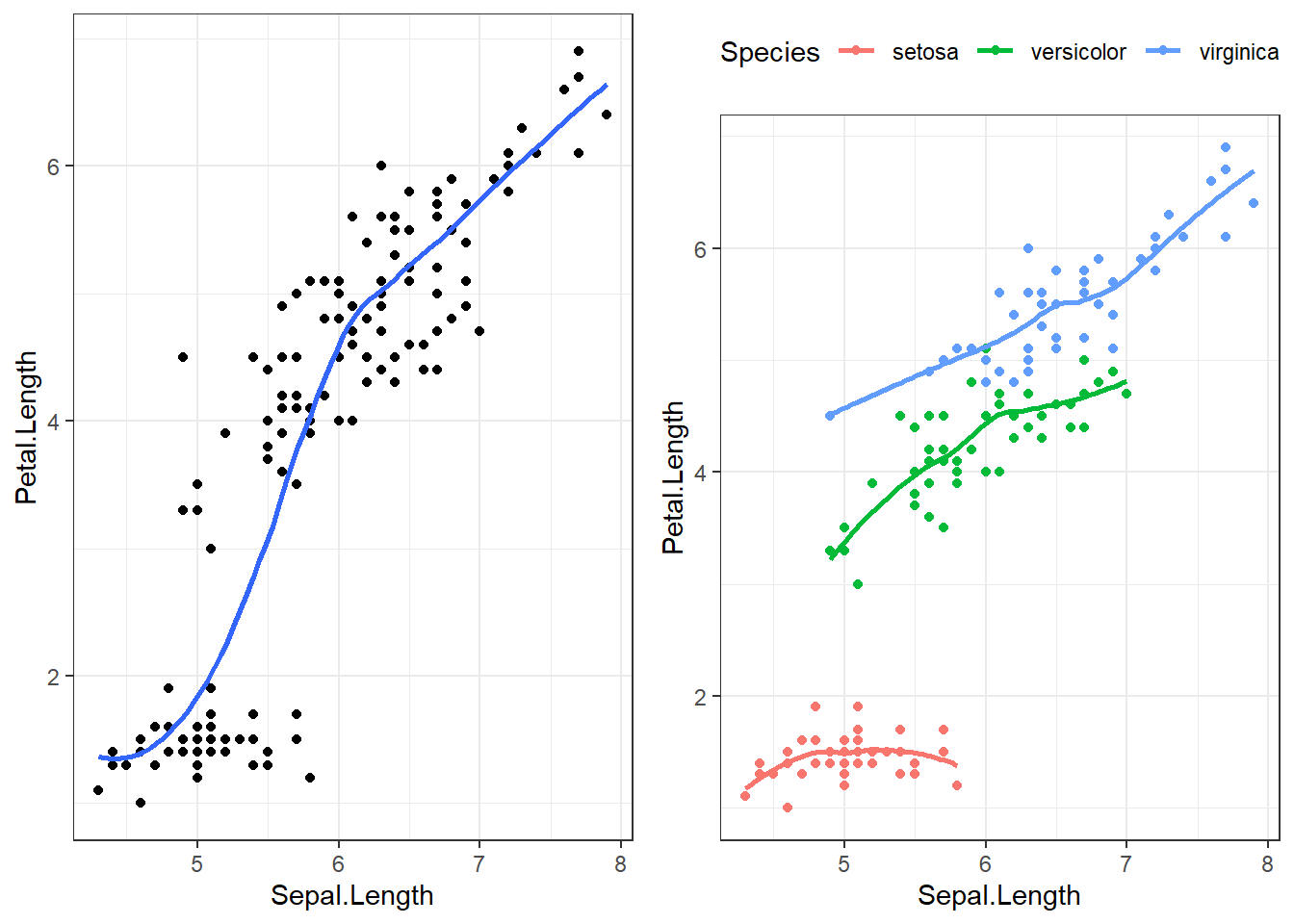## 8.1 Moderation

Moderation occurs when the relationship between two variables depends on a third variable.

• The third variable is referred to as the moderating variable or simply the moderator.
• The moderator affects the direction and/or strength of the relationship between the explanatory ($$x$$) and response ($$y$$) variable.
• This tends to be an important
• When testing a potential moderator, we are asking the question whether there is an association between two constructs, but separately for different subgroups within the sample.
• This is also called a stratified model, or a subgroup analysis.

### 8.1.1 Example 1: Simpson’s Paradox

Sometimes moderating variables can result in what’s known as Simpson’s Paradox. This has had legal consequences in the past at UC Berkeley.

### 8.1.2 Example 2: Sepal vs Petal Length in Iris flowers

Let’s explore the relationship between the length of the sepal in an iris flower, and the length (cm) of it’s petal.

overall <- ggplot(iris, aes(x=Sepal.Length, y=Petal.Length)) +
geom_point() + geom_smooth(se=FALSE) +
theme_bw()

by_spec <- ggplot(iris, aes(x=Sepal.Length, y=Petal.Length, col=Species)) +
geom_point() + geom_smooth(se=FALSE) +
theme_bw() + theme(legend.position="top")

gridExtra::grid.arrange(overall, by_spec , ncol=2)The points are clearly clustered by species, the slope of the lowess line between virginica and versicolor appear similar in strength, whereas the slope of the line for setosa is closer to zero. This would imply that petal length for Setosa may not be affected by the length of the sepal.